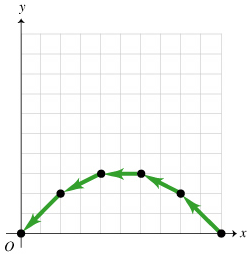# Problem: For the motion diagram given (Figure 1), sketch the shape of a possible graph for the y velocity versus time, vy(t). Use the indicated coordinate system. One unit of time elapses between consecutive dots in the motion diagram.

###### FREE Expert Solution

vy experiences constant acceleration. Velocity decreases from positive to zero before changing direction.

89% (256 ratings)###### Problem Details

For the motion diagram given (Figure 1), sketch the shape of a possible graph for the y velocity versus time, vy(t). Use the indicated coordinate system. One unit of time elapses between consecutive dots in the motion diagram.